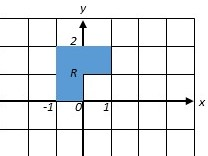# Calculate the double integral \iint_R(xy - y^3)dA for the following region R.

## Question:

Calculate the double integral {eq}\iint_R(xy - y^3) \, dA {/eq} for the following region R.## Properties of Area Integrals:

The area integral {eq}\iint_R f(x,y) \, dA {/eq} over regions of the plane has the following properties:

1) The linearity property:

{eq}\displaystyle \iint_R \left[af(x,y)+bg(x,y)\right] \, dA=a \iint_R f(x,y) \, dA + b \iint_R g(x,y) \, dA \, . {/eq}

2) The subdivision property: if {eq}R {/eq} can be split into smaller regions {eq}R_1,R_2 {/eq} which only intersect along their boundaries, then

{eq}\displaystyle \iint_R f(x,y) \, dA = \iint_{R_1} f(x,y) \, dA + \iint_{R_2} f(x,y) \, dA \, . {/eq}

The linearity property is occasionally useful, but in practice it is often better to use linearity properties of one-dimensional integrals. The subdivision property is frequently useful for breaking integrals over geometrically complicated regions up into integrals over geometrically simpler regions.

## Answer and Explanation:

We'll break the region {eq}R {/eq} up into two rectangles. The rectangle {eq}R_1 {/eq} has the Cartesian coordinate bounds {eq}-1 \le x \le 1 {/eq}, {eq}1 \le y \le 2 {/eq}: that is, {eq}R_1 {/eq} is the top portion of {eq}R {/eq}. The rectangle {eq}R_2 {/eq} has the Cartesian coordinate bounds {eq}-1 \le x \le 0 {/eq}, {eq}0 \le y \le 1 {/eq}: that is, {eq}R_2 {/eq} is the remaining bottom square in {eq}R {/eq}.

Now, we'll integrate over each of these areas separately. First,

{eq}\begin{align*} \iint_{R_1} (xy-y^3) \, dA&=\int_{x=-1}^1\int_{y=1}^2 (xy-y^3) \, dy \, dx&\text{(rewriting the area integral as an iterated integral)}\\ &=\int_{x=-1}^1\left(\frac{1}{2}xy^2-\frac{1}{4}y^4\right|_{y=1}^2 \, dx&\text{(evaluating the }y\text{-integral)}\\ &=\int_{x=-1}^1\left(\frac{1}{2}x(2^2)-\frac{1}{4}2^4-\frac{1}{2}x(1^2)+\frac{1}{4}1^4)\right) \, dx\\ &=\int_{-1}^1 \left(2x-4-\frac{1}{2}x+\frac{1}{4}\right) \, dx\\ &=\int_{-1}^1 \left(\frac{3}{2}x-\frac{15}{4}\right) \, dx\\ &=\left(\frac{3}{4}x^2-\frac{15}{4}x\right|_{-1}^0&\text{(evaluating the }x\text{-integral)}\\ &=\frac{3}{4}(1^2)-\frac{15}{4}(1)-\frac{3}{4}(-1)^2+\frac{15}{4}(-1)\\ &=\frac{3}{4}-\frac{15}{4}-\frac{3}{4}-\frac{15}{4}\\ &=-\frac{15}{2} \, . \end{align*} {/eq}

Next,

{eq}\begin{align*} \iint_{R_2} (xy-y^3) \, dA&=\int_{x=-1}^0\int_{y=0}^1 (xy-y^3) \, dy \, dx&\text{(rewriting the area integral as an iterated integral)}\\ &=\int_{x=-1}^0\left(\frac{1}{2}xy^2-\frac{1}{4}y^4\right|_{y=0}^1 \, dx&\text{(evaluating the }y\text{-integral)}\\ &=\int_{x=-1}^0\left(\frac{1}{2}x(1^2)-\frac{1}{4}1^4-\frac{1}{2}x(0^2)+\frac{1}{4}0^4)\right) \, dx\\ &=\int_{-1}^0 \left(\frac{1}{2}x-\frac{1}{4}\right) \, dx\\ &=\left(\frac{1}{4}x^2-\frac{1}{4}x\right|_{-1}^0&\text{(evaluating the }x\text{-integral)}\\ &=\frac{1}{4}(0^2)-\frac{1}{4}(0)-\frac{1}{4}(-1)^2+\frac{1}{4}(-1)\\ &=-\frac{1}{4}-\frac{1}{4}\\ &=-\frac{1}{2} \, . \end{align*} {/eq}

So we can use the subdivision property to compute the integral over the entire region:

{eq}\begin{align*} \iint_R (xy-y^3) \, dA&=\int_{R_1}(xy-y^3) \, dA + \int_{R_2} (xy-y^3) \, dA&\text{(by the subdivision property)}\\ &=-\frac{15}{2}-\frac{1}{2}&\text{(by the above calculations)}\\ &=-8 \, . \end{align*} {/eq}

So we've shown that {eq}\boxed{\iint_R (xy-y^3) \, dA = -8 \, .} {/eq}

#### Learn more about this topic:Double Integrals & Evaluation by Iterated Integrals

from GRE Math: Study Guide & Test Prep

Chapter 15 / Lesson 4
401